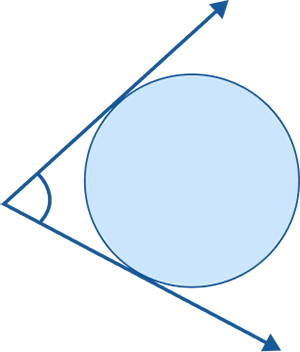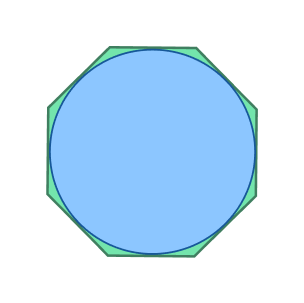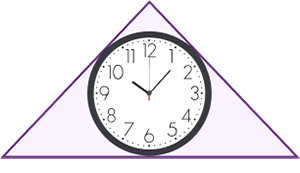# Circumscribe in Math: Definition with Examples

## What Does Circumscribed Mean in Math?

A circumscribed shape means a shape drawn around another shape such that it is touching it at points (vertices) but not cutting it.

When a shape is restricted within another shape and there is no way for the inside figure to pass through the outer figure, then we say that the inner shape is circumscribed by the outer shape. The outer shape touches the maximum number of points of the shape inside without crossing it.

To circumscribe means to restrict someone’s or something’s freedom. The prefix “circum” means “around,” and “inscribe” means “to be within the boundaries of.”  Thus, “circumscribed” means a shape is drawn around another shape.

Example 1: Circumscribed hexagon

Here, a hexagon is drawn around a circle touching the maximum number of points on the circumference but not crossing it.

Example 2: Circumscribed circle or circumcircle

Here, a circle is drawn around a triangle such that the circle passes through all the three vertices of the triangle. This is termed the circumcircle of a triangle.

## Circumscribe: Definition

Circumscribe means to draw a shape around another shape such that the outer shape touches maximum points of the inside shape but not crossing it such that the inside shape is enclosed or restricted within the bounds of the outer shape.

## Circumscribed Circle

The circle that passes through all the vertices of another geometrical figure or a polygon but does not cross it is called “circumscribed circle” or “circumcircle.”

When a circle circumscribes a quadrilateral, the quadrilateral is called a “cyclic quadrilateral.” The sum of the opposite angles of a cyclic quadrilateral equals 180 degrees.

Take a look at a square circumscribed by a circle. Here, the diameter of the square equals the diagonal of the square.

Diagonal of a square $= \sqrt{2}$ × (side of the square) = $\sqrt{2}$ × s

Thus, the diameter of the circle $= \sqrt{2}$ × (side of a square)

Using this formula, you can determine the side of the square if the diameter of the circle is given.

Circumcircle radius = Radius of the circle $= \frac{\sqrt{2} \times (side \;of \;a \;square)}{\sqrt{2}} = \frac{side\; of\; the\; square}{\sqrt{2}}$

## Steps to Construct a Circumcircle of a Triangle

Let’s understand the steps for constructing a circumcircle of a triangle.

Step 1: Construct a triangle with the required angle measurements.

Step 2: Draw perpendicular bisectors of two sides of the triangle.

Step 3: Label their point of intersection as O. Using O as the center and keeping OC as distance, draw a circle around the triangle touching all the three vertices or corners of the triangle.

## Circumscribed Triangle

A circumscribed triangle is a triangle that is drawn around another geometric shape in such a way that it touches the inside shape at maximum points without ever cutting it. Here, the triangle is the outside shape.

The triangle in the above figure surrounds the circle in such a way that its sides are tangent to the circle. We can also term it as a circle inscribed in a triangle.

## Circumscribed Polygon

A circumscribed polygon surrounds a geometric shape in a way that all of its vertices touch the boundaries of the polygon (regular or irregular).

A circumscribed quadrilateral encloses a geometric figure in such a way that its sides pass through maximum points of the inside shape without crossing it.

The figure below shows a quadrilateral enclosing a circle. Here, the sides of the quadrilateral act as a tangent to the circle. Note that not all quadrilaterals can be circumscribed.

When a square circumscribes a circle, the diameter of the circle equals the side of the square.

Here, the square ABCD circumscribes the circle with radius a such that the side of the square = 2a.

## Circumscribed Hexagon

A circumscribed hexagon surrounds a geometric figure so that it passes through each vertex of the inside shape.

In the given figure, a hexagon circumscribes a circle.

## Circumscribed Angle

When the arms of an angle touch the circle forming two tangents, the angle is said to be a circumscribed angle.

## Circumscribed vs. Inscribed

A geometrical shape drawn inside another shape such that all its vertices touch the outer shape without crossing it, the inside shape is called inscribed shape. A circumscribed figure is a shape drawn outside another shape.

We have to first understand which figure we want to describe before using the terms circumscribed and inscribed.

In the left image, we have an inscribed circle of a triangle.

(Note: We can also call this a circumscribed triangle.)

In the right image, we have the circumcircle, the circle circumscribes a triangle.

(Note: We can also call this an inscribed triangle.)

• Circumscribing involves placing one shape around another so that the outer shape touches maximum points of the inside shape.
• They can be found in many geometric figures like triangles and circles.
• The circumscribed circle of a polygon is the smallest circle that contains all its vertices.

## Conclusion

In this article, we learned about different circumscribed shapes. Let’s solve a few examples and practice problems for better understanding.

## Solved Examples on Circumscribed Shapes

1. A square circumscribes a circle with radius of 10 units. What is the area of the square?

Solution:

The square circumscribes a circle.

Radius of circle = 10 units

Diameter of circle = 20 units

Diameter of circle = Side of square = 20 units

Area of square = side2= 202 = 400 square units

2. The quadrilateral ABCD is encircled by a circle, as indicated in the illustration. Find the missing angles.

Solution:

The sum of the opposite sides of a quadrilateral enclosed in a circle equals 180 degrees.

Therefore,

∠A + ∠C = 180°

82°+∠C = 180°

∠C = 180° − 82°

∠C =98°

Similarly,

∠B +∠D = 180°

∠100°+∠D = 180°

∠D = 180°−∠100°

∠D = 80°

As a result, C = 102° and D = 75°.

## Practice Problems on Circumscribed Shapes

1

### When a circle is drawn outside a figure in a manner that it is passing through all the vertices of the figure but not intersecting it, then it is called _________.

circumscribed circle
circumscribe triangle
circumscribed polygon
circumscribed rectangle
CorrectIncorrect
A circumscribed circle is a circle drawn around a geometrical figure.
2

### Which option correctly describes the given diagram?a circle
inscribed angle
circumscribed angle
right angle
CorrectIncorrect
The angle whose arms are tangents to a circle is known as the circumscribed angle.
3

### The given diagram represents acircumscribed polygon
circumscribed pentagon
circumcircle
Inscribed octagon
CorrectIncorrect
This is a circumscribed polygon (octagon). It circumscribes a circle.
4

### What is the meaning of circumscribed shape?

A shape drawn around another shape
A shape drawn inside another shape
Both A and B
None
CorrectIncorrect
Correct answer is: A shape drawn around another shape
Circumscribe refers to drawing a shape around another shape such that the outer shape touches the corner points of the inside shape but not crossing it.
5

### The fancy clock shown below represents acircumcircle
circumscribed triangle
Triangle within a circle
circumscribed clock
CorrectIncorrect